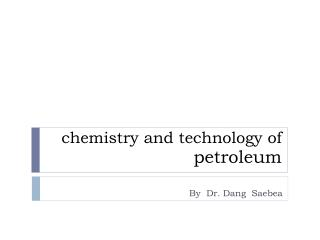DownloadDownload Presentationchemistry and technology of petroleum

# chemistry and technology of petroleum

Télécharger la présentation## chemistry and technology of petroleum

- - - - - - - - - - - - - - - - - - - - - - - - - - - E N D - - - - - - - - - - - - - - - - - - - - - - - - - - -
##### Presentation Transcript

1. chemistry and technology of petroleum By Dr. Dang Saebea

2. Example • A vacuum residue of Conradson carbon (wt% CCR=15)is fed into a flexicokingat a rate of 200,000 lb/h, of API=8.5 and with a sulphur content of 3.0 wt%. • Find the amount of yield (lb/h) and their sulphur content. • Calculate yield of liquid products in BPD.

3. Yield Correlations for Flexicoking The yield correlations for flexicoking are based on the Conradson carbon content of the vacuum residue (CCR, wt%), its API gravity and sulphur content (Sf). Data compiled by Maples (1993) are correlated to express weight percent yields. Gas composition:

4. Yield Correlations for Flexicoking Sulphur distribution in products: Gravity of flexicoker feed and gas oil

5. Solution

6. Solution

7. Calculate yield of liquid products in BPD. Gas oil density = gas oil SG x water density = 0.95 x 350.28 lb/barrel = 332.766 lb/barrel Gas oil (BPD) = 115,828 lb/hr x24 hr/day /332.766 lb/barrel = 8353.8 BPD

8. Example 100 m3/h of heavy naphtha (HN) with specific gravity of 0.778 has the following composition: A =11.5 vol%, N = 21.7 vol% and P= 66.8 vol% is to be reformed to naphtha reformate of RON =94. Calculate the yields of each product for that reformer.

9. Reformer correlations RONF=research octane number of feed; RONR=research octane number of reformate;C+5vol% =volume percent of reformate yield; SCFB H2=standard cubic foot of H2 produced/barrel of feed; K =characterization factor (TB)1/3/SG; TB= absolute mid-boiling of feed, (˚R); SG =specific gravity of feed; N = napthenesvol % and A ผ aromatics vol %

10. Solution Given RONR= 94 and N+2A=44.7%, gives the following reformer yield: C+5 vol% =142.7914 – 0.77033x(94) + 0.219122x(44.7) = 80.17% The yields for the other products can then be calculated from the correlations in Table .

11. Solution The material balance for the reformer is presented in the following table:

12. The End